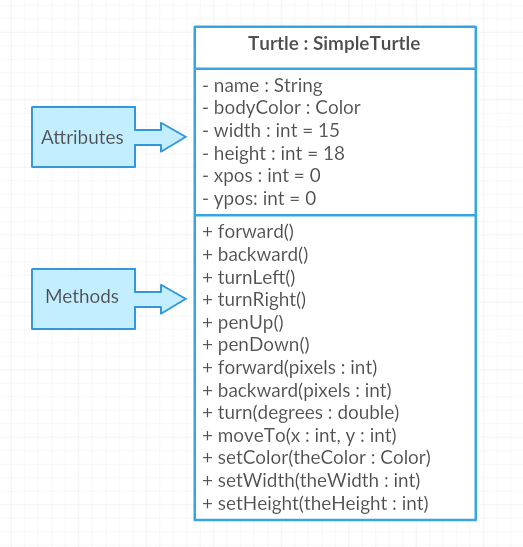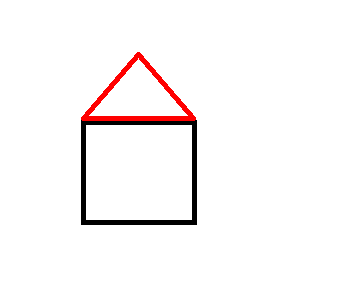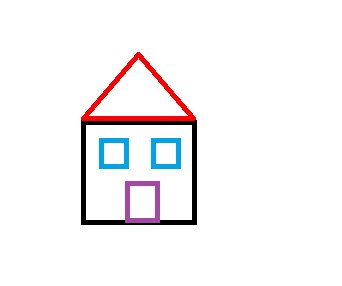# 2.4. Calling Methods With Parameters¶

In the last lesson, we used methods like forward() and turnRight() to make the turtle draw lines. You may have noticed that forward() and backward() always move the same number of pixels (100 pixels), and turnRight() and turnLeft() always turn at right angles (90 degrees). This is a little limiting. What if we wanted to draw a triangle or the letter A? These require smaller angles to draw diagonal lines and different length lines.

Luckily, there are more complex methods in the Turtle class that let you specify the number of pixels to move forward or the number of degrees to turn. For example, we can use `forward(25)` to make the turtle go forward 25 pixels or `turn(30)` to make the turtle turn 30 degrees. The methods signatures define formal parameters, which are placeholders for values that will be passed into the method when it is called. The values passed in are arguments or actual parameters.

Although some people use the words parameters and arguments interchangeably, there is a subtle difference. When a method is defined, the method signature will list the formal parameters. The method implementation will use the formal parameters to customize the object behavior. The code below shows the signature for the forward method, which has a parameter variable called pixels (the method implementation is not shown). The subsequent code block shows the method call forward(25). The actual parameter value 25 will get copied into the formal parameter variable pixels.

```// The method signature has one formal parameter of type int.
public void forward(int pixels) // parameter pixels
```
```// Method call must pass an argument (also called actual parameter) that is an int value
yertle.forward(25);
```Check your understanding

The Turtle class inherits many attributes and methods from another class called SimpleTurtle. You will learn about inheritance in a later lesson. But for now you can look at the Turtle class diagram listed in the figure below that shows some (not all) of the attributes and methods inherited from the SimpleTurtle class. The formal parameters are shown between the parentheses that follow the method name, and list the type after the parameter name. While the notation might be different that what you are used to for Java code, the class model should give you some ideas about new ways to move your turtle objects. You can see all the methods that are inherited in Turtle in this javadoc (documentation) file.Figure 1: Turtle Class Diagram

Methods are said to be overloaded when there are multiple methods with the same name but a different number or type of parameters. For example, the Turtle class diagram lists two different forward methods, one with no parameters and one that has a formal parameter of type int that tells the turtle how much to move forward.

Note

A class diagram may list the parameter type after the variable name, such as `pixels : int`. However, when you write Java code you always list the type before the variable `int pixels`.

Experiment with using some turtle methods that take parameters. If the code below does not work in your browser, you can also use the Turtle code at this repl.it link (refresh page after forking and if it gets stuck) or download the files here to use in your own IDE.

1. Can you make yertle draw a square and change the pen color for each side of the square? Try something like: yertle.setColor(Color.red); This uses the Color class in Java which has some colors predefined like red, yellow, blue, magenta, cyan. You can also use more specific methods like setPenColor, setBodyColor, and setShellColor. 2. Can you draw a triangle? The turnRight() method always does 90 degree turns, but you’ll need 60 degree angles for a equilateral triangle. Use the turn method which has a parameter for the angle of the turn in degrees. For example, turn(90) is the same as turnRight(). Try drawing a triangle with different colors.Test your understanding - mixed up code

Try the following mixed up code to draw a simple house made of a square and a triangle roof.The following code uses a turtle to draw a simple house, but the lines are mixed up. Note that the turtle variable name is “builder” rather than “yertle” or “myrtle”. Drag the code blocks to the right and put them in the correct order to first draw a square for the house and then a red triangle for the roof. Click on the “Check Me” button to check your solution. You can copy and paste this code in the Active Code window above to see it in action.

## 2.4.1.Programming Challenge : Turtle House¶Design a house and have the turtle draw it with different colors below (or with this repl.it link). Can you add windows and a door?

To draw a window, you will need to do penUp() to walk the turtle into position. For example, given a turtle named “builder”:

```builder.penUp();
builder.moveTo(120,200);
builder.penDown();
```

It may help to act out the code pretending you are the turtle. Remember that the angles you turn depend on which direction you are facing, and the turtle begins facing up.

## 2.4.2. Summary¶

• Methods define the behaviors or functions for objects.

• To use an object’s method, you must use the object name and the dot (.) operator followed by the method name, for example object.method();

• Some methods take parameters/arguments that are placed inside the parentheses object.method(arguments).

• Values provided in the parameter list need to correspond to the order and type in the method signature.